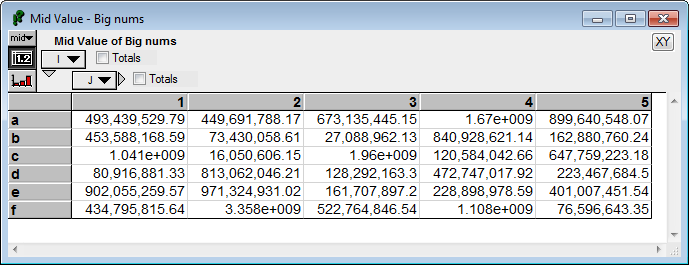# CellNumberFormat

(diff) ← Older revision | Latest revision (diff) | Newer revision → (diff)

New to Analytica 5.0

## CellNumberFormat( format, digits, showZeroes, thousandsSeparators, currency, dateFormat, fullPrecision )

Used to change the number format of a table cell from within a Computed cell formats in the Cell Format Expression attribute. All parameters are optional, only the components that are being changed need to specified. The parameters here are the same as the parameters of NumberToText.

### Parameters

«format»
The type of format used for numbers (but not date or date/time values). It can be one of the following values (or just the first letter):
"Suffix" (e.g., 39.63K)
"Exponential" (e.g., 3.963e+004)
"Fixed Point" (e.g., 39632.55)
"Integer" (e.g., 39633)
"Percent" (e.g., 3963254.89%)
"Date" (e.g., 4-Jul-2012) -- forces numbers to display as dates
"Boolean"  (e.g., True)
"Hexadecimal" (e.g., 0x9ad1)
"Binary" (e.g., 0b1001101011010001)
«digits»
The number of significant digits for Suffix and Exponential formats, or the number of digits to the right of the decimal point for Fixed Point and Percent formats.
«showZeroes»
Boolean specifying whether to show trailing zeroes so that all numbers have the same number of decimal digits, even if they are zero.
«separators»
Boolean (0 or 1) controls whether to show separators. In the US, these are commas between each group of three digits (e.g., 39,632.55).
«currency»
Specifies the currency symbol to display, or the symbol and its placement relative to the digits and sign. It recognizes these templates, where $ can be replaced by any currency symbol: "$-#"
"$#-" "-$#"
"-#$" "#$-"
"#-$" "(#$)"
"($#)" "regional" «dateFormat» The template used to display dates, times, or date-time numbers. It substitutes these patterns, and anything else is printed literally. "SHORT", "ABBREV", "LONG", "TIME", "DATETIME": regional-specific standard formats. y, yy, yyyy: The year. y = two digits, no leading zero. yy = two digits. yyyy = four digits. M, MM: The month number, without or with leading zero. MMM, MMMM: The month name, abbreviated or full ("Jan" or "January") d, dd: The day number, without or with leading zero. w: The weekday number www, wwww: The weekday name, abbreviated or full ("Wed", or "Wednesday") q: The quarter (1 thru 4) h, hh: The numeric hour from 1 thru 12, without or with leading zero. tt: AM or PM H, HH: The numeric hour from 0 thru 23, without or with leading zero. m, mm: The numeric number of minutes, from 0 to 59, without or with leading zero. s, ss: The whole number portion of seconds, from 0 to 59, without or with leading zero. .ssssss: The fractional seconds (up to 6 digits) ' literal text ': The literal text is shown. If you want the ' character to show, then you must double it. «fullPrecision» A boolean value. When set to true, the number is displayed at full precision even if this requires exceeding the number of digits specified in «digits». ## Examples Only the first number in each column should be formatted as currency, and there may be a varying number of non-numbers before the first number. If Cumulate(IsNumber(Self),I)=1 Then CellNumberFormat(currency: '$-#' )

:Numbers below 1B use fixed point, above that exponential.

If Abs(Self)<1B
Then CellNumberFormat( 'Fixed Point', thousandsSeparators:true )
Else CellNumberFormat('Exponential')

: